# 图像处理流水线

1. 采集和存储存储：需要捕获图像（例如使用相机）并将其作为文件（例如 JPEG 文件）存储在某些设备（例如硬盘）上。

2. 加载到内存并保存到磁盘：图像需要从磁盘读取到内存并使用某些数据结构（例如，numpy ndarray）存储，并且数据结构需要在以后序列化到图像文件中，可能是在对图像运行一些算法之后。

3. 操作、增强和恢复：我们需要运行一些预处理算法来完成以下工作：

• 在图像上运行一些转换（采样和操作；例如，灰度转换）
• 增强图像质量（过滤；例如，去模糊）
• 恢复图像不受噪声影响
4. 分割：为了提取感兴趣的对象，需要对图像进行分割。

5. 信息提取/表示：图像需要以某种替代形式表示；例如，以下选项之一：

• 可以从图像中计算一些手工制作的特征描述符（例如，使用经典图像处理的 HOG 描述符）
• 一些特征可以从图像中自动学习（例如，通过深度学习在神经网络的隐藏层中学习的权重和偏差值）
• 将使用该替代表示法 来表示图像
6. 图像理解/解释此表示将用于通过以下内容更好地理解图像：

• 图像分类（例如，图像是否包含人体对象）
• 对象识别例如在带有边框的图像中查找汽车对象的位置）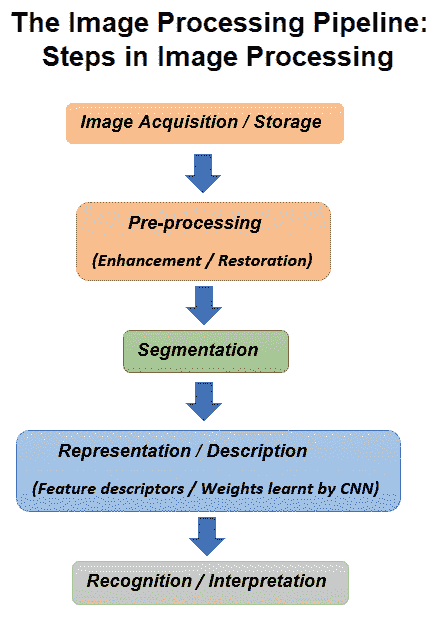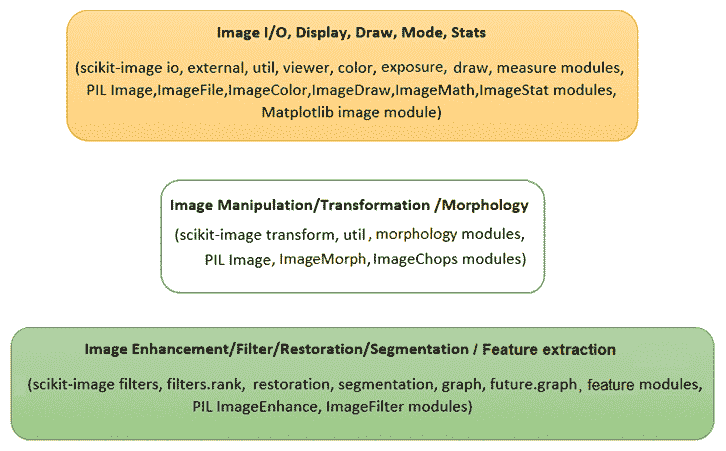• scipy.ndimageopencv用于不同的图像处理任务
• scikit-learn用于经典机器学习
• tensorflowkeras用于深度学习

# 安装 pip

***# 在 Python 中安装一些图像处理库

matplotlib库主要用于显示，而numpy将用于存储图像。scikit-learn库将用于建立用于图像处理的机器学习模型，scipy库将主要用于图像增强。scikit-imagemahotasopencv库将用于不同的图像处理算法。

# 安装 Anaconda 配线架

More about installing Anaconda for different OSes can be found at https://conda.io/docs/user-guide/install/index.html.

# 使用 Python 进行图像 I/O 和显示

# for inline image display inside notebook
# % matplotlib inline
import numpy as np
from PIL import Image, ImageFont, ImageDraw
from PIL.ImageChops import add, subtract, multiply, difference, screen
import PIL.ImageStat as stat
from skimage import color, viewer, exposure, img_as_float, data
from skimage.transform import SimilarityTransform, warp, swirl
from skimage.util import invert, random_noise, montage
import matplotlib.image as mpimg
import matplotlib.pylab as plt
from scipy.ndimage import affine_transform, zoom
from scipy import misc



# 使用 PIL 读取、保存和显示图像

PIL 函数open()Image对象中的磁盘读取图像，如下代码所示。图像作为PIL.PngImagePlugin.PngImageFile类的对象加载，我们可以使用宽度、高度和模式等属性来查找图像的大小（宽度x高度像素或图像分辨率）和模式：

im = Image.open("../images/parrot.png") # read the image, provide the correct pathprint(im.width, im.height, im.mode, im.format, type(im))# 453 340 RGB PNG <class 'PIL.PngImagePlugin.PngImageFile'>im.show() # display the image


# 使用 Matplotlib 读取、保存和显示图像

im = mpimg.imread("../images/hill.png")  # read the image from disk as a numpy ndarrayprint(im.shape, im.dtype, type(im))      # this image contains an α channel, hence num_channels= 4# (960, 1280, 4) float32 <class 'numpy.ndarray'>plt.figure(figsize=(10,10))plt.imshow(im) # display the imageplt.axis('off')plt.show()# 使用 Matplotlib imshow（）显示时进行插值

Matplotlib 的imshow()函数提供了许多不同类型的插值方法来绘制图像。当要打印的图像很小时，这些函数特别有用。让我们使用下图所示的小 50 x 50lena图像来查看使用不同插值方法绘制的效果：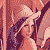im = mpimg.imread("../images/lena_small.jpg") # read the image from disk as a numpy ndarray
methods = ['none', 'nearest', 'bilinear', 'bicubic', 'spline16', 'lanczos']
fig, axes = plt.subplots(nrows=2, ncols=3, figsize=(15, 30),
subplot_kw={'xticks': [], 'yticks': []})
for ax, interp_method in zip(axes.flat, methods):
ax.imshow(im, interpolation=interp_method)
ax.set_title(str(interp_method), size=20)
plt.tight_layout()
plt.show()# 使用 scikit 图像读取、保存和显示图像

im = imread("../images/parrot.png") # read ...


# 使用 scikit image 的宇航员数据集

im = data.astronaut()
imshow(im), show()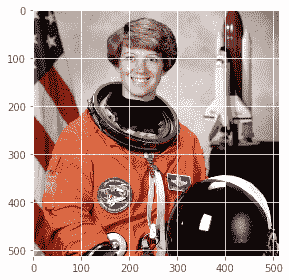# 使用 scipy misc 读取、保存和显示图像

scipymisc模块也可用于图像 I/O 和显示。以下各节演示如何使用misc模块功能。

# 使用 scipy.misc 的人脸数据集

im = misc.face() # load the raccoon's face imagemisc.imsave('face.png', im) # uses the Image module (PIL)plt.imshow(im), plt.axis('off'), plt.show()im = misc.imread('../images/pepper.jpg')print(type(im), im.shape, im.dtype)# <class 'numpy.ndarray'> (225, 225, 3) uint8


# 从一种文件格式转换为另一种文件格式

im = Image.open("../images/parrot.png")print(im.mode)  #  RGBim.save("../images/parrot.jpg")


im = Image.open("../images/hill.png")print(im.mode)# RGBAim.convert('RGB').save("../images/hill.jpg") # first convert to RGB mode


# 图像类型（模式）

• 单通道图像每个像素由单个值表示：
• 二进制（单色）图像（每个像素由单个 0-1 位表示）
• 灰度图像（每个像素可以用 8 位表示，其值通常在 0-255 之间）
• 多通道图像每个像素由一组值表示：
• 三通道图像；例如，以下各项：
• RGB 图像每个像素由三个元组（r、g、b值表示，表示每个像素的红色、绿色和蓝色通道颜色值。
• HSV 图像每个像素由三个元组（h、s、v值表示，分别表示色调（颜色）、饱和度（颜色与白色的混合程度）和（亮度与黑色的混合程度）每个像素的通道颜色值。HSV 模型以类似于人眼感知颜色的方式描述颜色
• 四通道图像；例如，RGBA 图像每个像素由三个元组（r、g、b、α值表示，最后一个通道表示透明度。

# 从一种图像模式转换为另一种图像模式

im = imread("images/parrot.png", as_gray=True)print(im.shape)#(362L, 486L)


im = imread("../images/Ishihara.png")im_g = color.rgb2gray(im)plt.subplot(121), plt.imshow(im, ...


# 某些颜色空间（通道）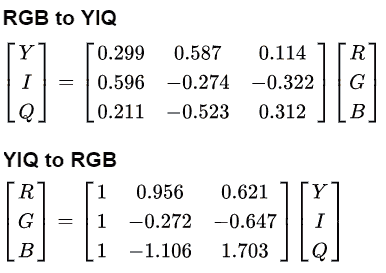# 从一个颜色空间转换到另一个颜色空间

im = imread("../images/parrot.png")im_hsv = color.rgb2hsv(im)plt.gray()plt.figure(figsize=(10,8))plt.subplot(221), plt.imshow(im_hsv[...,0]), plt.title('h', size=20), plt.axis('off')plt.subplot(222), plt.imshow(im_hsv[...,1]), plt.title('s', size=20), plt.axis('off')plt.subplot(223), plt.imshow(im_hsv[...,2]), plt.title('v', size=20), plt.axis('off')plt.subplot(224), plt.axis('off')plt.show()


# 转换图像数据结构

im = Image.open('../images/flowers.png') # read image into an Image object with PILim = np.array(im) # create a numpy ndarray from the Image objectimshow(im) # use skimage imshow to display the imageplt.axis('off'), show()im = imread('../images/flowers.png') ...


# 基本图像处理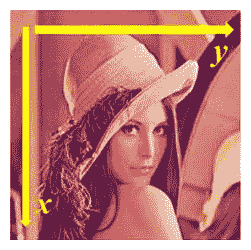# 基于 numpy 阵列切片的图像处理

lena = mpimg.imread("../images/lena.jpg") # read the image from disk as a numpy ndarrayprint(lena[0, 40])# [180  76  83]# print(lena[10:13, 20:23,0:1]) # slicinglx, ly, _ = lena.shapeX, Y = np.ogrid[0:lx, 0:ly]mask = (X - lx / 2) ** 2 + (Y - ly / 2) ** 2 > lx * ly / 4lena[mask,:] = 0 # masksplt.figure(figsize=(10,10))plt.imshow(lena), plt.axis('off'), plt.show()# 简单图像变形-使用交叉溶解的两幅图像的α-混合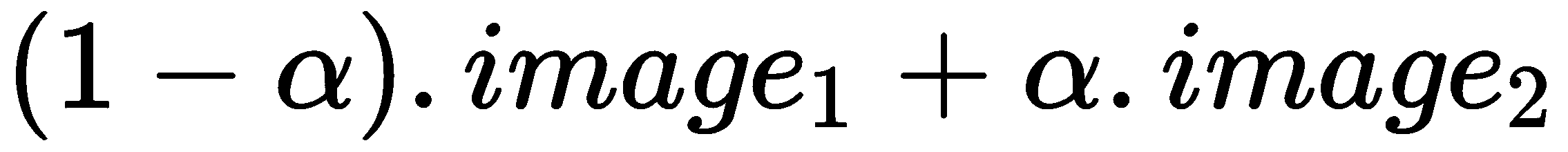im1 = mpimg.imread("../images/messi.jpg") / 255 # scale RGB values in [0,1]
i = 1
plt.figure(figsize=(18,15))
for alpha in np.linspace(0,1,20):
plt.subplot(4,5,i)
plt.imshow((1-alpha)*im1 + alpha*im2)
plt.axis('off')
i += 1
plt.show()# 基于 PIL 的图像处理

PIL 为我们提供了许多操作图像的功能；例如，使用点变换更改像素值或对图像执行几何变换。让我们首先加载 parrot PNG 图像，如下代码所示：

im = Image.open("../images/parrot.png")        # open the image, provide the correct pathprint(im.width, im.height, im.mode, im.format) # print image size, mode and format# 486 362 RGB PNG


# 裁剪图像

im_c = im.crop((175,75,320,200)) # crop the rectangle given by (left, top, right, bottom) from the image
im_c.show()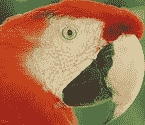# 调整图像大小

im = Image.open("../images/clock.jpg")print(im.width, im.height)# 107 105im.show()# 否定图像

im = Image.open("../images/parrot.png")
im_t = im.point(lambda x: 255 - x)
im_t.show()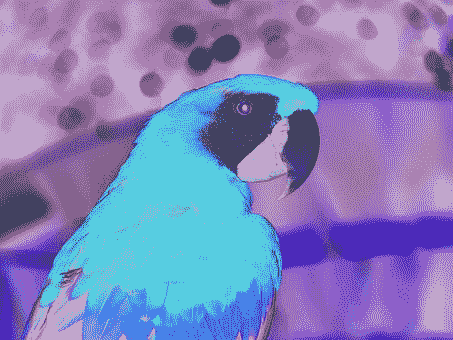# 将图像转换为灰度

im_g = im.convert('L')   # convert the RGB color image to a grayscale image


# 一些灰度变换

im_g.point(lambda x: 255*np.log(1+x/255)).show()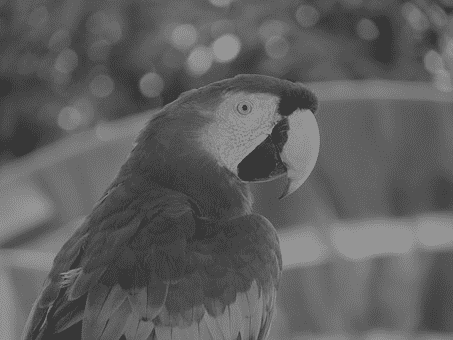im_g.point(lambda x: 255*(x/255)**0.6).show()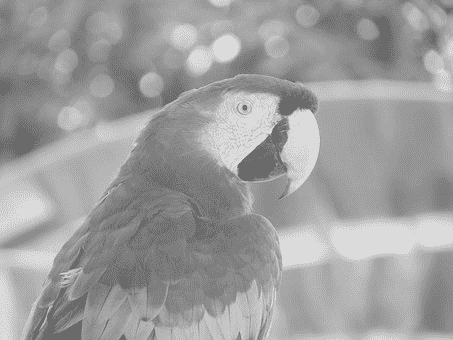# 一些几何变换

im.transpose(Image.FLIP_LEFT_RIGHT).show() # reflect about the vertical axis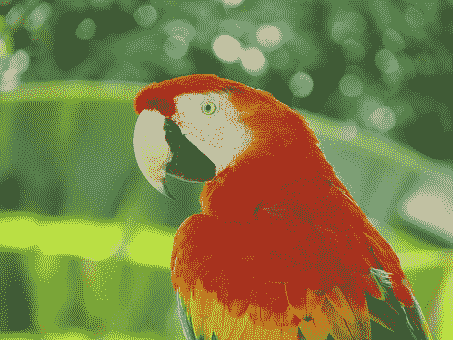# 更改图像的像素值

# choose 5000 random locations inside image
im1 = im.copy() # keep the original image, create a copy
n = 5000
x, y = np.random.randint(0, im.width, n), np.random.randint(0, im.height, n)
for (x,y) in zip(x,y):
im1.putpixel((x, y), ((0,0,0) if np.random.rand() < 0.5 else (255,255,255))) # salt-and-pepper noise
im1.show()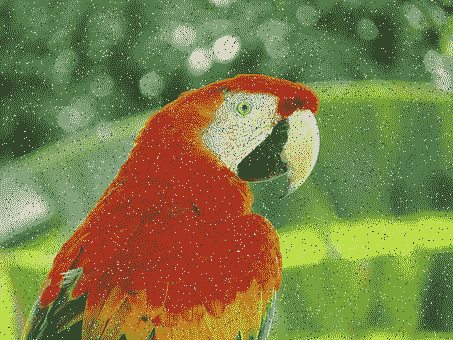# 画图

im = Image.open("../images/parrot.png")draw = ImageDraw.Draw(im)draw.ellipse((125, 125, 200, 250), fill=(255,255,255,128))del drawim.show()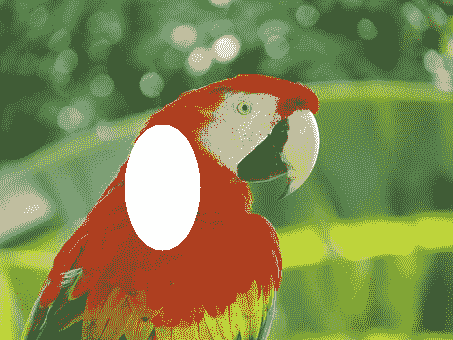# 在图像上绘制文本

draw = ImageDraw.Draw(im)
font = ImageFont.truetype("arial.ttf", 23) # use a truetype font
draw.text((10, 5), "Welcome to image processing with python", font=font)
del draw
im.show()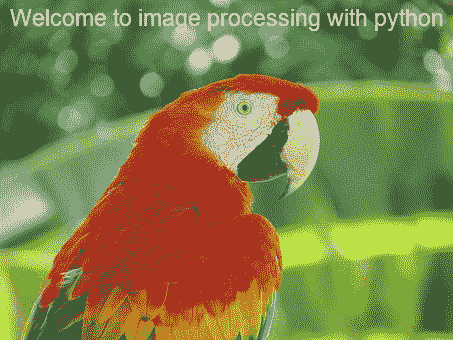# 创建缩略图

im_thumbnail = im.copy() # need to copy the original image firstim_thumbnail.thumbnail((100,100))# now paste the thumbnail on the image im.paste(im_thumbnail, (10,10))
im.save("../images/parrot_thumb.jpg")
im.show()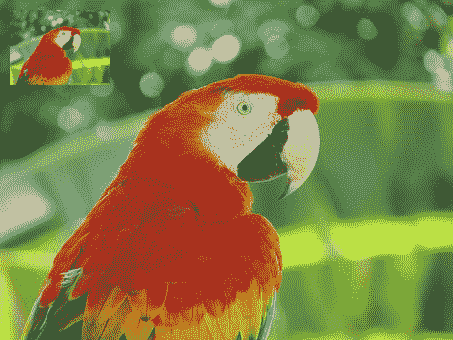# 计算图像的基本统计信息

s = stat.Stat(im)
print(s.extrema) # maximum and minimum pixel values for each channel R, G, B
# [(4, 255), (0, 255), (0, 253)]
print(s.count)
# [154020, 154020, 154020]
print(s.mean)
# [125.41305674587716, 124.43517724970783, 68.38463186599142]
print(s.median)
# [117, 128, 63]
print(s.stddev)
# [47.56564506512579, 51.08397900881395, 39.067418896260094]


# 绘制图像 RGB 通道的像素值直方图

histogram()函数可用于计算每个通道像素的直方图（像素值与频率的表格），并返回串联输出（例如，对于 RGB 图像，输出包含3 x 256=768值）：

pl = im.histogram()plt.bar(range(256), pl[:256], color='r', alpha=0.5)plt.bar(range(256), pl[256:2*256], color='g', alpha=0.4)plt.bar(range(256), pl[2*256:], color='b', alpha=0.3)plt.show()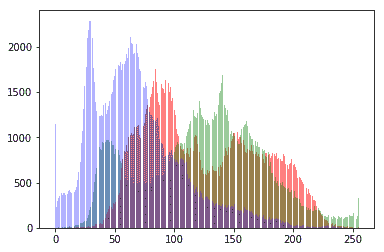# 分离图像的 RGB 通道

ch_r, ch_g, ch_b = im.split() # split the RGB image into 3 channels: R, G and B
# we shall use matplotlib to display the channels
plt.figure(figsize=(18,6))
plt.subplot(1,3,1); plt.imshow(ch_r, cmap=plt.cm.Reds); plt.axis('off')
plt.subplot(1,3,2); plt.imshow(ch_g, cmap=plt.cm.Greens); plt.axis('off')
plt.subplot(1,3,3); plt.imshow(ch_b, cmap=plt.cm.Blues); plt.axis('off')
plt.tight_layout()
plt.show() # show the R, G, B channels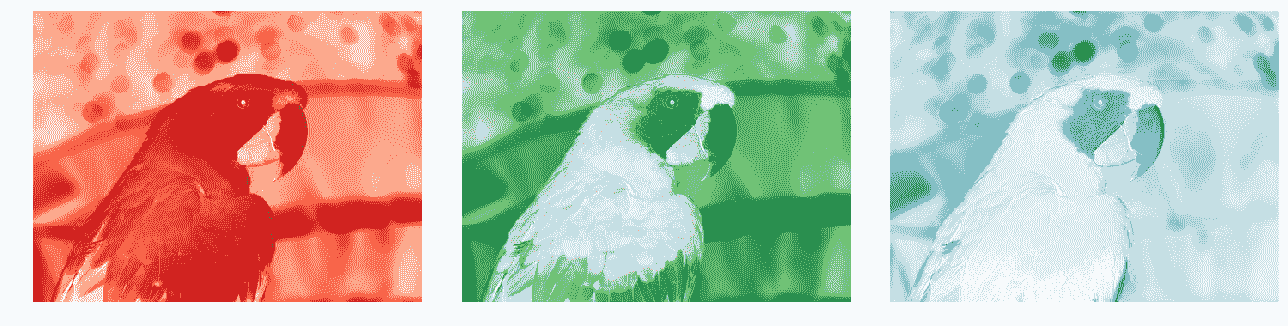# 组合图像的多个通道

im = Image.merge('RGB', (ch_b, ch_g, ch_r)) # swap the red and blue channels obtained last time with split()im.show()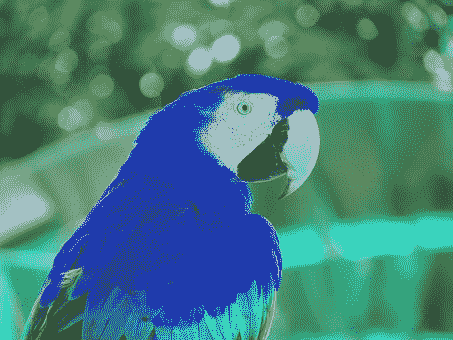# α-混合两幅图像

blend()函数可用于通过使用常数α插值两个给定图像（大小相同）来创建新图像。两个图像必须具有相同的大小和模式。输出图像如下所示：

out=图像1（1.0-α）+图像2α

im1 = Image.open("../images/parrot.png")
im2 = Image.open("../images/hill.png")
# 453 340 1280 960 RGB RGBA
im1 = im1.convert('RGBA') # two images have different modes, must be converted to the same mode
im2 = im2.resize((im1.width, im1.height), Image.BILINEAR) # two images have different sizes, must be converted to the same size
im = Image.blend(im1, im2, alpha=0.5).show()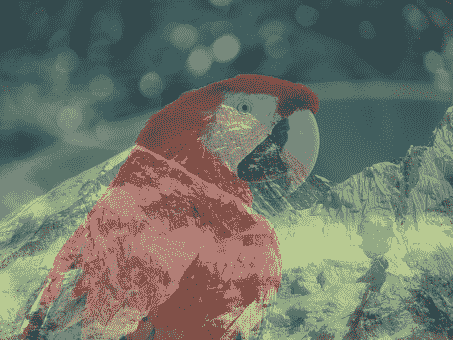# 叠加两幅图像

im1 = Image.open("../images/parrot.png")im2 = Image.open("../images/hill.png").convert('RGB').resize((im1.width, im1.height))multiply(im1, im2).show()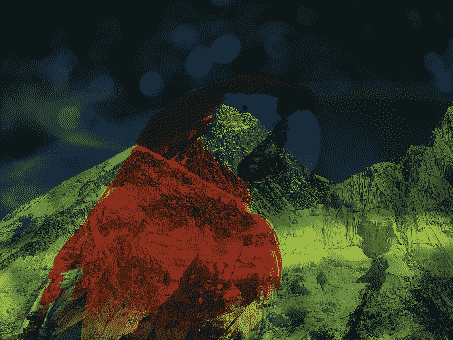# 添加两个图像

add(im1, im2).show()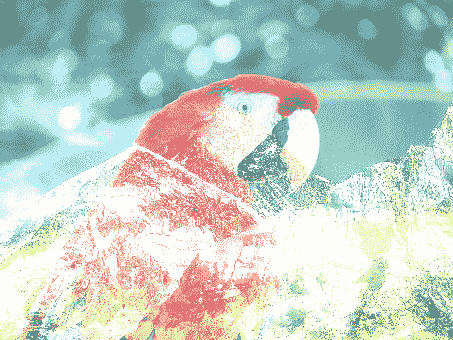# 计算两幅图像之间的差异

from PIL.ImageChops import subtract, multiply, screen, difference, addim1 = Image.open("../images/goal1.png") # load two consecutive frame images from the videoim2 = Image.open("../images/goal2.png")im = difference(im1, im2)im.save("../images/goal_diff.png")plt.subplot(311)plt.imshow(im1)plt.axis('off')plt.subplot(312)plt.imshow(im2)plt.axis('off')plt.subplot(313) ...


# 减去两幅图像并叠加两幅图像负片

subtract()功能可用于首先减去两幅图像，然后将结果除以比例（默认为 1.0）并添加偏移量（默认为 0.0）。类似地，screen()功能可用于将两个反转图像叠加在彼此的顶部。

# 使用 warp（）函数进行反向扭曲和几何变换

scikit imagetransform模块的warp()函数可用于图像几何变换的反向扭曲（在上一节中讨论），如以下示例所示。

im = imread("../images/parrot.png")
tform = SimilarityTransform(scale=0.9, rotation=np.pi/4,translation=(im.shape/2, -100))
warped = warp(im, tform)
import matplotlib.pyplot as plt
plt.imshow(warped), plt.axis('off'), plt.show()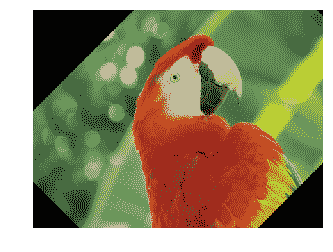# 应用漩涡变换

im = imread("../images/parrot.png")swirled = swirl(im, rotation=0, strength=15, radius=200)plt.imshow(swirled)plt.axis('off')plt.show()


# 向图像中添加随机高斯噪声

im = img_as_float(imread("../images/parrot.png"))
plt.figure(figsize=(15,12))
sigmas = [0.1, 0.25, 0.5, 1]
for i in range(4):
noisy = random_noise(im, var=sigmas[i]**2)
plt.subplot(2,2,i+1)
plt.imshow(noisy)
plt.axis('off')
plt.title('Gaussian noise with sigma=' + str(sigmas[i]), size=20)
plt.tight_layout()
plt.show()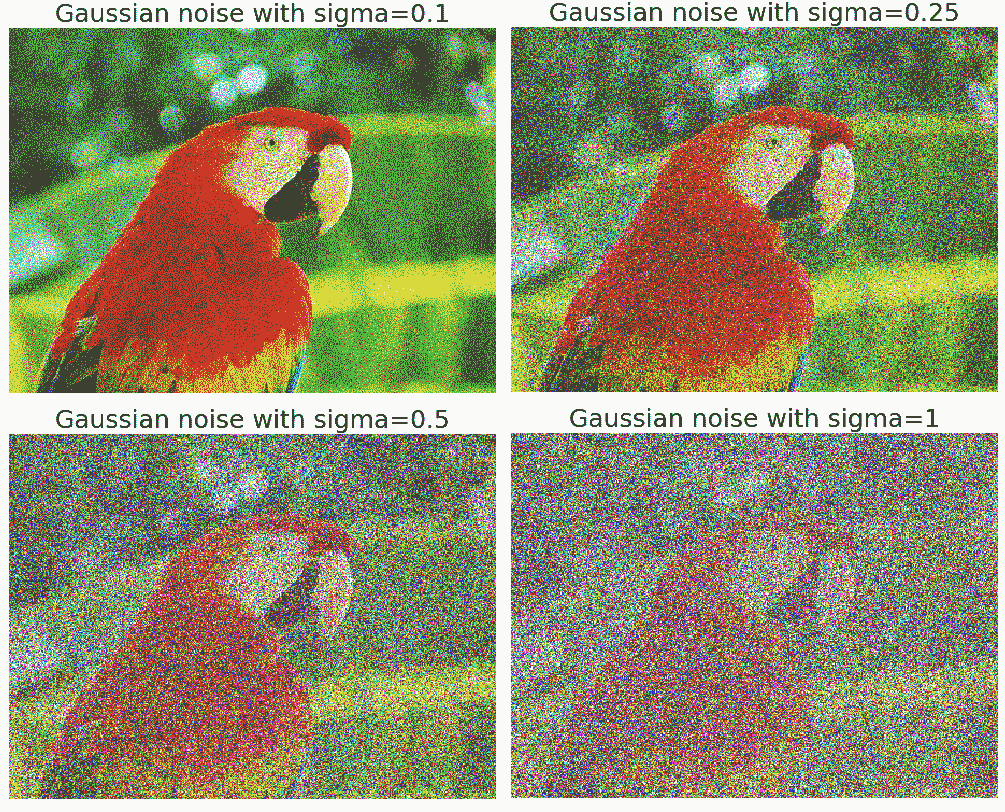# 用 Matplotlib 进行图像处理

*# 为图像绘制轮廓线

im = rgb2gray(imread("../images/einstein.jpg")) # read the image from disk as a numpy ndarrayplt.figure(figsize=(20,8))plt.subplot(131), plt.imshow(im, cmap='gray'), plt.title('Original Image', size=20) plt.subplot(132), plt.contour(np.flipud(im), colors='k', levels=np.logspace(-15, 15, 100))plt.title('Image Contour Lines', size=20)plt.subplot(133), plt.title('Image Filled Contour', size=20), plt.contourf(np.flipud(im), cmap='inferno')plt.show()


# 问题

1. 使用scikit-image库的函数读取图像集合并将其显示为蒙太奇。
2. 使用scipy ndimagemisc模块的功能对图像进行缩放、裁剪、调整大小和应用仿射变换。
3. 创建 Gotham Instagram 过滤器的 Python 翻拍版（https://github.com/lukexyz/CV-Instagram-Filters （提示：使用 PILsplit()merge()numpy interp()功能操作图像以创建通道插值（https://www.youtube.com/watch?v=otLGDpBglEA &功能=播放器（嵌入）。
4. 使用 scikit image 的warp()功能实现漩涡变换。注意，swirl变换也可以用以下等式表示：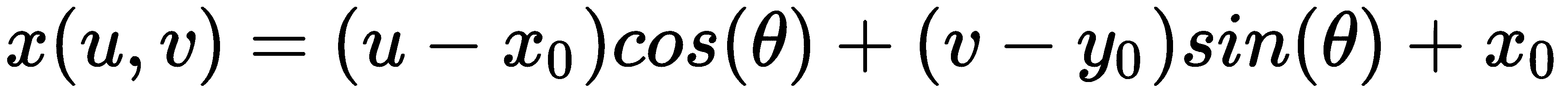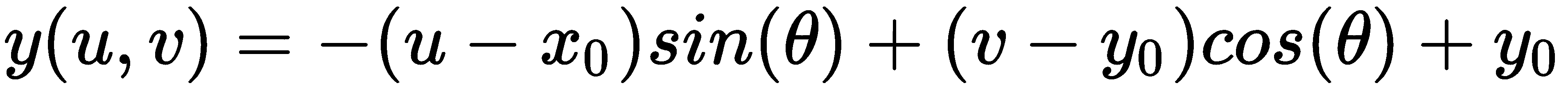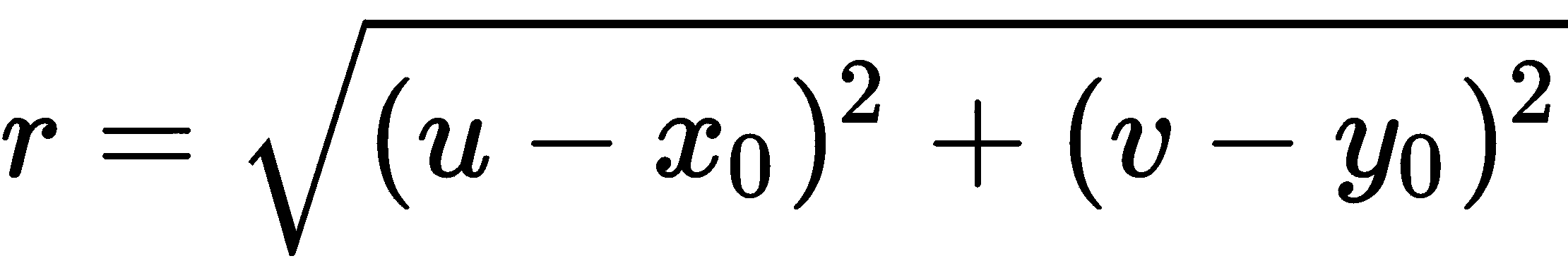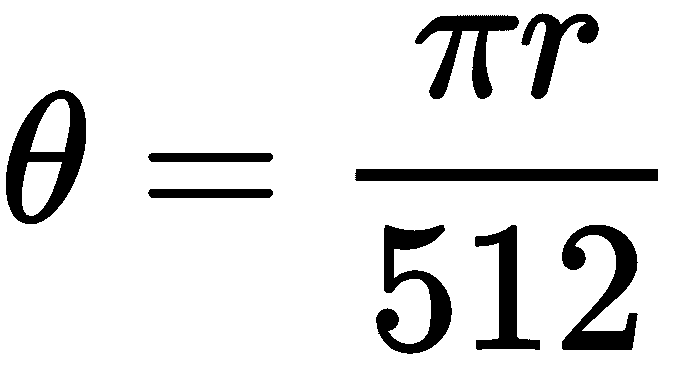1. 执行以下给出的波形变换（提示：使用 scikit 图像的warp()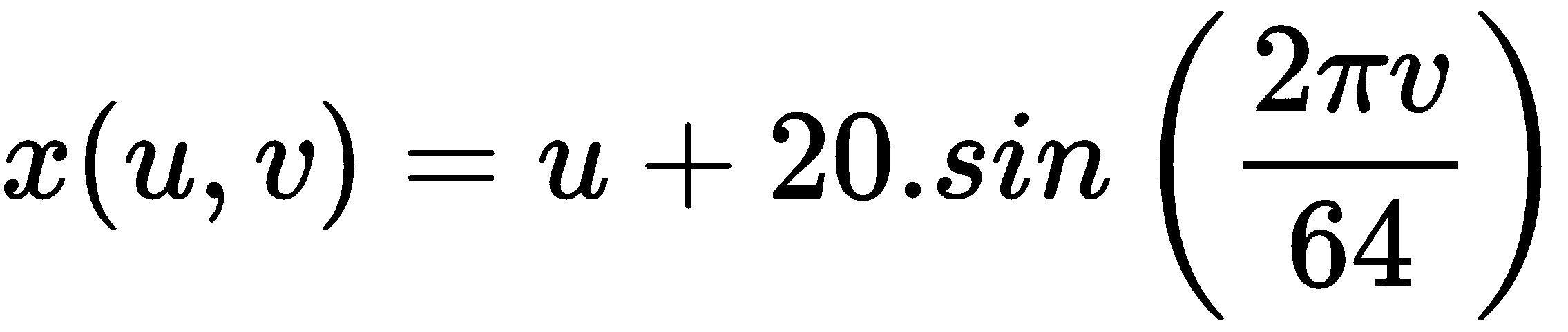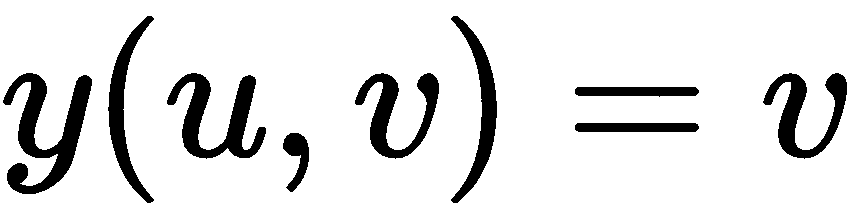1. 使用 PIL 加载带有调色板的 RGB.png文件，并将其转换为灰度图像。此问题摘自此帖：https://stackoverflow.com/questions/51676447/python-use-pil-to-load-png-file-gives-strange-results/51678271#51678271 。通过索引调色板，将以下 RGB 图像（来自VOC2012数据集）转换为灰度图像：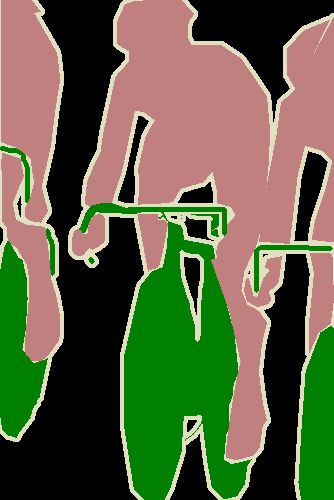1. 为本章中使用的鹦鹉图像的每个颜色通道绘制 3D 图（提示：使用mpl_toolkits.mplot3d模块的plot_surface()功能和 NumPy 的meshgrid()功能）。

2. 使用 SCIKIT 图像的 To.T0.模块的莱娜 T1 来估计从源到目标图像的单应矩阵，并使用 AUT2 T2 函数嵌入在空白画布中的图像（如下所示）：

| 输入图像 | 输出图像 | |||